$$\gdef\badDispatch#1{\textbf{\textcolor{e1432d}{#1}}} \gdef\noKatexForm#1{\badDispatch{#1}} \gdef\largeDot{\raisebox{0.06em}{\tiny∙}} \gdef\rarrbar{ {\raisebox{-0.05em}{→}\mkern{-0.13em}{\large\shortmid}}} \gdef\larrbar{ { {\large\shortmid}\mkern{-0.13em}{\raisebox{-0.05em}{←}}}} \gdef\suptrans{^\mathsf{T}} \gdef\supdagger{^\dagger} \gdef\rawsymbol#1{\operatorname{#1}} \gdef\colorsymbol#1#2{\textcolor{#1}{\rawsymbol{#2}}} \gdef\lsymbol#1{\colorsymbol{262626}{#1}} \gdef\msymbol#1{\colorsymbol{b50800}{#1}} \gdef\osymbol#1{\colorsymbol{00427f}{#1}} \gdef\lstring#1{\textcolor{666666}{\textrm{\textquotedblleft{#1}\textquotedblright}}} \gdef\boldForm#1{\textbf{#1}} \gdef\lrform#1{ {\textcolor{e1432d}{#1}}} \gdef\lgform#1{ {\textcolor{4ea82a}{#1}}} \gdef\lbform#1{ {\textcolor{3e81c3}{#1}}} \gdef\rform#1{ {\textcolor{e1432d}{#1}}} \gdef\gform#1{ {\textcolor{4ea82a}{#1}}} \gdef\bform#1{ {\textcolor{3e81c3}{#1}}} \gdef\rgform#1{ {\textcolor{dc841a}{#1}}} \gdef\gbform#1{ {\textcolor{47a5a7}{#1}}} \gdef\rbform#1{ {\textcolor{c74883}{#1}}} \gdef\waform#1{ {\textcolor{6b6b6b}{#1}}} \gdef\wbform#1{ {\textcolor{929292}{#1}}} \gdef\wcform#1{ {\textcolor{c5c5c5}{#1}}} \gdef\barToken{\mathbf{|}} \gdef\filledRectangleToken{▮} \gdef\emptyRectangleToken{▯} \gdef\filledSquareToken{■} \gdef\emptySquareToken{□} \gdef\filledToken{∙} \gdef\emptyToken{∘} \gdef\cardinalRewrite#1#2{\rewrite{#1}{#2}} \gdef\primed#1{ {#1}^{\prime}} \gdef\tinybullet{ {\tiny●}} \gdef\forwardFactor{\uparrow} \gdef\backwardFactor{\downarrow} \gdef\neutralFactor{\mid} \gdef\arrowhead{⏵} \gdef\deSymbol{ { { {\raisebox{1.1pt}{\tinybullet}\mkern{-1pt}{→}}}\,}} \gdef\ueSymbol{ {\raisebox{1pt}{\tinybullet\raisebox{-0.03em}{\mkern{-0.3pt}{\tiny──}\mkern{-0.3pt}}\tinybullet}\,}} \gdef\ldeSymbol{ { { {\raisebox{1.1pt}{\tinybullet}\mkern{-1pt}{\longrightarrow}}}\,}} \gdef\lueSymbol{ {\raisebox{1pt}{\tinybullet\raisebox{-0.03em}{\mkern{-0.3pt}{\tiny────}\mkern{-0.3pt}}\tinybullet}\,}} \gdef\de#1#2{ { {#1} \deSymbol {#2}}} \gdef\ue#1#2{ { {#1} \ueSymbol {#2}}} \gdef\tde#1#2#3{ { {#1} \overset{#3}{\deSymbol} {#2}}} \gdef\tue#1#2#3{ { {#1} \overset{#3}{\ueSymbol} {#2}}} \gdef\ltde#1#2#3{ { {#1} \overset{#3}{\ldeSymbol} {#2}}} \gdef\ltue#1#2#3{ { {#1} \overset{#3}{\lueSymbol} {#2}}} \gdef\mapsfrom{\htmlClass{hreflect}{\mapsto}} \gdef\longmapsfrom{\htmlClass{hreflect}{\longmapsto}} \gdef\diffd{𝕕} \gdef\partialdof#1{\partial {#1}} \gdef\emptySet{\empty} \gdef\unknown{\wbform{\text{?}}} \gdef\notApplicable{\wbform{\text{---}}} \gdef\textAnd{\text{\,and\,}} \gdef\identicallyEqualSymbol{\equiv} \gdef\congruentSymbol{\equiv} \gdef\isomorphicSymbol{\cong} \gdef\homotopicSymbol{\simeq} \gdef\approxEqualSymbol{\approx} \gdef\bijectiveSymbol{\cong} \gdef\defeq{\;≝\;} \gdef\defEqualSymbol{\;≝\;} \gdef\tailEqualSymbol{\underdot{=}} \gdef\headEqualSymbol{\dot{=}} \gdef\ruledelayed{:\to} \gdef\mathdollar{\text{\textdollar}} \gdef\hyphen{\text{-}} \gdef\endash{\text{--}} \gdef\emdash{\text{---}} \gdef\updownarrows{\uparrow\!\downarrow} \gdef\vthinspace{\mkern{1pt}} \gdef\dlq{\text{\textquotedblleft}} \gdef\drq{\text{\textquotedblright}} \gdef\dprime{ {\prime\prime}} \gdef\inverse#1{ { {#1}^{-1}}} \gdef\inverseSymbol{\inverse{□}} \gdef\groupDirectProduct#1{#1} \gdef\groupDirectProductSymbol{\times} \gdef\groupInverse{\inverse} \gdef\groupPower#1#2{ {#1}^{#2}} \gdef\groupCommutator#1#2{\left[{#1},{#2}\right]} \gdef\groupoidInverse{\inverse} \gdef\latticeBFS#1{\textrm{bfs}({#1})} \gdef\pathHomomorphism#1{#1} \gdef\groupoidFunction#1{#1} \gdef\groupoidHomomorphism#1{#1} \gdef\affineModifier#1{\overrightharpoon{#1}} \gdef\supercirc#1{#1^{\circ}} \gdef\supercircb#1{#1\!\degree} \gdef\toroidalModifier#1{\supercirc{#1}} \gdef\modulo#1{\supercirc{#1}} \gdef\dividesSymbol{\,|\,} \gdef\groupFunction#1{#1} \gdef\groupHomomorphism#1{#1} \gdef\automorphisms{\operatorname{Aut}} \gdef\endomorphisms{\operatorname{End}} \gdef\transportMap#1{\transportMapSymbol_{#1}} \gdef\transportMapSymbol{\tau} \gdef\action#1{#1} \gdef\selfAction#1{\hat{#1}} \gdef\actionGroupoid#1{\utilde{#1}} \gdef\cardinalGroup#1{G^*({#1})} \gdef\signed#1{ {#1}^*} \gdef\transportAtlas#1{T_{#1}} \gdef\inverted#1{\underline{#1}} \gdef\mirror#1{\overline{#1}} \gdef\pathComposeSymbol{ {\,∷\,}} \gdef\pathCompose#1#2{ {#1}\pathComposeSymbol{#2}} \gdef\translateSymbol{\uparrow} \gdef\backwardTranslateSymbol{\downarrow} \gdef\pathTranslate#1#2{ {#1}\,\translateSymbol\,{#2}} \gdef\pathLeftTranslate#1{ {#1}{\,\translateSymbol}} \gdef\pathBackwardTranslate#1#2{ {#1}{\,\backwardTranslateSymbol\,}{#2}} \gdef\pathLeftBackwardTranslate#1{ {#1}{\,\backwardTranslateSymbol}} \gdef\compactCovariantDifference#1#2{\Delta_{#1}\,{#2}} \gdef\covariantDifference{ {#1}\,\Delta\,{#2}} \gdef\forwardDifference{\Delta^{+}} \gdef\backwardDifference{\Delta^{-}} \gdef\centralDifference{\Delta} \gdef\pathForwardDifference#1{\forwardDifference_{#1}} \gdef\pathBackwardDifference#1{\backwardDifference_{#1}} \gdef\pathCentralDifference#1{\centralDifference_{#1}} \gdef\pathHead#1{\pathHeadVector{#1}} \gdef\pathTail#1{\pathTailVector{#1}} \gdef\pathHeadVector#1{ {#1}^{\bullet}} \gdef\pathTailVector#1{ {#1}_{\bullet}} \gdef\pathReverse#1{ {#1}^{\dagger}} \gdef\pathIntegral#1#2{ {#1} \int {#2}} \gdef\pathIntegralSymbol{ {\int}} \gdef\pathDot#1#2{ {#1} \cdot {#2}} \gdef\pathDotSymbol{ {\cdot}} \gdef\compactBasis#1{\mathscr{B}} \gdef\length{\operatorname{len}} \gdef\signedLength{\operatorname{len^*}} \gdef\andFn{\operatorname{and}} \gdef\orFn{\operatorname{or}} \gdef\notFn{\operatorname{not}} \gdef\vertexList{\operatorname{vertices}} \gdef\vertexList{\operatorname{vertices}} \gdef\edgeList{\operatorname{edges}} \gdef\pathList{\operatorname{paths}} \gdef\cardinalList{\operatorname{cards}} \gdef\signedCardinalList{\operatorname{cards^*}} \gdef\wordOf{\operatorname{word}} \gdef\headVertex{\operatorname{head}} \gdef\tailVertex{\operatorname{tail}} \gdef\basis{\operatorname{basis}} \gdef\split{\operatorname{split}} \gdef\lcm{\operatorname{lcm}} \gdef\minimalContractionSets{\operatorname{MCSets}} \gdef\minimalContractions{\operatorname{MC}} \gdef\grade{\operatorname{grade}} \gdef\support{\operatorname{support}} \gdef\coefficient{\operatorname{coeff}} \gdef\domain{\operatorname{domain}} \gdef\codomain{\operatorname{codomain}} \gdef\modFunction{\operatorname{mod}} \gdef\isPrime#1{#1\textrm{ prime}} \gdef\blank{\_} \gdef\emptyWord{} \gdef\multiwordSymbol#1{\mathbf{#1}} \gdef\wordSymbol#1{\mathtt{#1}} \gdef\word#1{#1} \gdef\pathMap#1{#1} \gdef\function#1{#1} \gdef\imageModifier#1{ {#1}^{\rightarrow}} \gdef\preimageModifier#1{ {#1}^{\leftarrow}} \gdef\multiImageModifier#1{ {#1}^{\Rightarrow}} \gdef\multiPreimageModifier#1{ {#1}^{\Leftarrow}} \gdef\functionComposition#1{#1} \gdef\functionCompositionSymbol{∘} \gdef\route#1#2#3{[{#1}\!:\!{#2}\!:\!{#3}]} \gdef\multiroute#1#2#3{[{#1}\!:\!{#2}\!:\!{#3}]} \gdef\pathWord#1#2#3{ {#1}\!:\!{#2}\!:\!{#3}} \gdef\nullPath{\bot} \gdef\nullElement{\bot} \gdef\path#1{#1} \gdef\vert#1{#1} \gdef\underdot#1{\underset{\raisebox{0.3em}{.}}{#1}} \gdef\tvert#1{\underdot{#1}} \gdef\hvert#1{\dot{#1}} \gdef\edge#1{#1} \gdef\card#1{\mathtt{#1}} \gdef\path#1{#1} \gdef\quiver#1{#1} \gdef\bindingRuleSymbol{\to} \gdef\compactBindingRuleSymbol{:} \gdef\cayleyQuiverSymbol#1{\selfAction{#1}} \gdef\bindCayleyQuiver#1#2{\selfAction{#1}[#2]} \gdef\bindActionQuiver#1#2{#1[#2]} \gdef\bindSize#1#2{#1(#2)} \gdef\bindCardSize#1#2{#1[#2]} \gdef\bindCards#1#2{#1[#2]} \gdef\subSize#1#2{#1_{#2}} \gdef\gridQuiver#1{\textrm{Grid}^{#1}} \gdef\treeQuiver#1{\textrm{Tree}^{#1}} \gdef\bouquetQuiver#1{\textrm{Bq}^{#1}} \gdef\lineQuiver{\textrm{L}} \gdef\cycleQuiver{\textrm{C}} \gdef\squareQuiver{\textrm{Sq}} \gdef\cubicQuiver{\textrm{Cbc}} \gdef\triangularQuiver{\textrm{Tri}} \gdef\hexagonalQuiver{\textrm{Hex}} \gdef\rhombilleQuiver{\textrm{Rmb}} \gdef\limit#1#2{\lim_{#2}\,#1} \gdef\realVectorSpace#1{\mathbb{R}^{#1}} \gdef\realVectorSpace#1{\mathbb{C}^{#1}} \gdef\matrixRing#1#2{M_{#2}(#1)} \gdef\groupoid#1{#1} \gdef\group#1{#1} \gdef\field#1{#1} \gdef\ring#1{#1} \gdef\indeterminate#1{#1} \gdef\semiring#1{#1} \gdef\sym#1{#1} \gdef\matrix#1{#1} \gdef\polynomial#1{#1} \gdef\polynomialRing#1#2{#1[{#2}]} \gdef\routeSymbol#1{#1} \gdef\multirouteSymbol#1{\mathbf{#1}} \gdef\planSymbol#1{\mathbf{#1}} \gdef\ringElement#1{#1} \gdef\matrixPart#1#2#3{#1\llbracket{#2,#3}\rrbracket} \gdef\matrixRowPart#1{#1} \gdef\matrixColumnPart#1{#1} \gdef\subMatrixPart#1#2#3{#1_{#2,#3}} \gdef\matrixDotSymbol{\cdot} \gdef\matrixPlusSymbol{+} \gdef\wordGroup#1{\wordGroupSymbol_{#1}} \gdef\wordGroupSymbol{\Omega} \gdef\wordRing#1{\wordRingSymbol_{#1}} \gdef\wordRingSymbol{\Omega\!\degree} \gdef\linearCombinationCoefficient#1{ {\textcolor{888888}{#1}}} \gdef\plan#1{#1} \gdef\planRing#1{\planRingSymbol_{#1}} \gdef\planRingSymbol{\Phi} \gdef\basisPath#1#2{\mathbf{#1}_{#2}} \gdef\basisPathWeight#1#2{ {#1}_{#2}} \gdef\unitSymbol{\mathbf{e}} \gdef\unitVertexField{\unitSymbol_1} \gdef\forwardSymbol{f} \gdef\backwardSymbol{b} \gdef\symmetricSymbol{s} \gdef\antisymmetricSymbol{a} \gdef\wordVector#1#2{\unitSymbol_{#1}^{#2}} \gdef\gradOf#1{\grad\,{#1}} \gdef\grad{\nabla} \gdef\divOf#1{\div\,{#1}} \gdef\div{\dot{\nabla}} \gdef\laplacianOf#1{\laplacian\,{#1}} \gdef\laplacian{\ddot{\nabla}} \gdef\suchThat#1#2{ {#1}|{#2}} \gdef\chart#1{\chartSymbol_{#1}} \gdef\chartSymbol{C} \gdef\graphRegionIntersectionSymbol{\cap} \gdef\graphRegionUnionSymbol{\cup} \gdef\pathIso{\simeq} \gdef\factorial#1{#1!} \gdef\power#1#2{ {#1}^{#2}} \gdef\repeatedPower#1#2{ {#1}^{#2}} \gdef\contractionLattice#1{\operatorname{Con}(#1)} \gdef\contractedRelation#1{\sim_{#1}} \gdef\isContracted#1#2{ {#1} \sim {#2}} \gdef\isContractedIn#1#2#3{ {#1} \sim_{#3} {#2}} \gdef\isNotContracted#1#2{ {#1} \not \sim {#2}} \gdef\isNotContractedIn#1#2#3{ {#1} \not \sim_{#3} {#2}} \gdef\contractionSum#1{#1} \gdef\contractionSumSymbol{\sqcup} \gdef\contractionProduct#1{#1} \gdef\contractionProductSymbol{ {\cdot}} \gdef\graph#1{#1} \gdef\graphHomomorphism#1{#1} \gdef\coversSymbol{\sqsupseteq} \gdef\coveredBySymbol{\sqsubseteq} \gdef\strictlyCoversSymbol{\sqsupset} \gdef\strictlyCoveredBySymbol{\sqsubset} \gdef\covering#1#2#3{ {#2} \sqsupseteq_{#1} {#3}} \gdef\powerSet#1{\powerSetSymbol({#1})} \gdef\powerSetSymbol{\mathscr{P}} \gdef\existsForm#1#2{\exists\,{#1}\,:\,{#2}} \gdef\forAllForm#1#2{\forall\,{#1}\,:\,{#2}} \gdef\notted#1{\notSymbol {#1}} \gdef\andSymbol{\land} \gdef\orSymbol{\lor} \gdef\notSymbol{\lnot} \gdef\latticeElement#1{#1} \gdef\latticeMeetSymbol{\wedge} \gdef\latticeJoinSymbol{\vee} \gdef\latticeTop{\top} \gdef\latticeBottom{\bot} \gdef\latticeGreaterSymbol{>} \gdef\latticeGreaterEqualSymbol{\ge} \gdef\latticeLessSymbol{<} \gdef\latticeLessEqualSymbol{\le} \gdef\grpname#1{\operatorname{\mathsf{#1}}} \gdef\mkg#1#2#3{\grpname{#1}({#2},{#3})} \gdef\mka#1#2#3{\mathfrak{#1}({#2},{#3})} \gdef\generalLinearAlgebra#1#2{\mka{gl}{#1}{#2}} \gdef\generalLinearGroup#1#2{\mkg{GL}{#1}{#2}} \gdef\specialLinearAlgebra#1#2{\mka{sl}{#1}{#2}} \gdef\specialLinearGroup#1#2{\mkg{SL}{#1}{#2}} \gdef\projectiveGeneralLinearAlgebra#1#2{\mka{pgl}{#1}{#2}} \gdef\projectiveGeneralLinearGroup#1#2{\mkg{PGL}{#1}{#2}} \gdef\projectiveSpecialLinearAlgebra#1#2{\mka{psl}{#1}{#2}} \gdef\projectiveSpecialLinearGroup#1#2{\mkg{PSL}{#1}{#2}} \gdef\orthogonalAlgebra#1#2{\mka{o}{#1}{#2}} \gdef\orthogonalGroup#1#2{\mkg{O}{#1}{#2}} \gdef\specialOrthogonalAlgebra#1#2{\mka{so}{#1}{#2}} \gdef\specialOrthogonalGroup#1#2{\mkg{SO}{#1}{#2}} \gdef\unitaryAlgebra#1{\mka{u}{#1}{#2}} \gdef\unitaryGroup#1{\mkg{U}{#1}{#2}} \gdef\specialUnitaryAlgebra#1#2{\mka{su}{#1}{#2}} \gdef\specialUnitaryGroup#1#2{\mkg{su}{#1}{#2}} \gdef\spinAlgebra#1#2{\mka{spin}{#1}{#2}} \gdef\spinGroup#1#2{\mkg{Spin}{#1}{#2}} \gdef\pinAlgebra#1#2{\mka{pin}{#1}{#2}} \gdef\pinGroup#1#2{\mkg{Pin}{#1}{#2}} \gdef\symmetricGroup#1{\grpname{Sym}({#1})} \gdef\presentation#1{#1} \gdef\groupPresentation#1#2{\left\langle\,{#1}\,\,\middle|\mathstrut\,\,{#2}\,\right\rangle} \gdef\groupRelationIso{=} \gdef\groupGenerator#1{#1} \gdef\groupRelator#1{#1} \gdef\groupElement#1{#1} \gdef\groupoidElement#1{#1} \gdef\iconstruct#1#2{ {#1}\,\,\middle|{\large\mathstrut}\,\,{#2}} \gdef\setConstructor#1#2{\left\{\,\iconstruct{#1}{#2}\,\right\}} \gdef\multisetConstructor#1#2{\left\{\mkern{-2.3pt}\left|\,\,\iconstruct{#1}{#2}\,\right|\mkern{-2.3pt}\right\}} \gdef\multisets#1{\mathscr{M}({#1})} \gdef\signedMultisets#1{\mathscr{M^*}({#1})} \gdef\setSymbol#1{#1} \gdef\multisetSymbol#1{#1} \gdef\setElementSymbol#1{#1} \gdef\multisetElementSymbol#1{#1} \gdef\multisetMultiplicitySymbol{\raisebox{.1em}{\small\#}\mkern{.1em}} \gdef\boundMultiplicityFunction#1{\operatorname{ {#1}^{\sharp}}} \gdef\constructor#1#2{\left.{#1}\,\,\middle|\mathstrut\,\,{#2}\right.} \gdef\elemOf#1#2{ { {#1} \in {#2} }} \gdef\notElemOf#1#2{ { {#1} \notin {#2} }} \gdef\edgeOf#1#2{ { {#1} {\in}_E {#2} }} \gdef\vertOf#1#2{ { {#1} {\in}_V {#2} }} \gdef\pathOf#1#2{ { {#1} {\in}_P {#2} }} \gdef\pathType#1{\operatorName{path}{#1}} \gdef\vertexType#1{\operatorName{vertex}{#1}} \gdef\edgeType#1{\operatorName{edge}{#1}} \gdef\multisetType#1{\operatorName{mset}{#1}} \gdef\signedMultisetType#1{\operatorName{mset^*}{#1}} \gdef\fromTo#1{#1} \gdef\fromToSymbol{\mapsto} \gdef\vertexCountOf#1{|{#1}|} \gdef\vertices#1{ V_{#1} } \gdef\edges#1{ E_{#1} } \gdef\vertexField#1{#1} \gdef\edgeField#1{#1} \gdef\pathVector#1{\mathbf{#1}} \gdef\pathVectorSpace#1{\mathscr{#1}} \gdef\baseField#1{#1} \gdef\finiteField#1{\mathbb{F}_{#1}} \gdef\functionSpace#1#2{#2^{#1}} \gdef\pathGroupoid#1{ { \Gamma_{#1} }} \gdef\forwardPathQuiver#1#2{ {\overrightharpoon{#1}_{#2}}} \gdef\backwardPathQuiver#1#2{ {\overrightharpoon{#1}^{#2}}} \gdef\pathQuiver#1{ {\overrightharpoon{#1}}} \gdef\mto#1#2{ {#1}\mapsto{#2}} \gdef\mtoSymbol{\mapsto} \gdef\groupWordRewriting#1{\langle{#1}\rangle} \gdef\rewrite#1#2{ {#1}\mapsto{#2}} \gdef\rewritingRule#1#2{ {#1}\mapsto{#2}} \gdef\rewritingSystem#1{\mathcal{#1}} \gdef\multiwayBFS#1{\textrm{bfs}({#1})} \gdef\rewritingStateBinding#1#2{ {\left.{#1}\!\mid\!{#2}\right.}} \gdef\rewritingRuleBinding#1#2{#1{\left[\,{#2}\,\right]}} \gdef\namedSystem#1{\mathsf{#1}} \gdef\genericRewritingSystem{\namedSystem{System}} \gdef\stringRewritingSystem{\namedSystem{Str}} \gdef\turingMachineRewritingSystem{\namedSystem{TM}} \gdef\cellularAutomatonRewritingSystem{\namedSystem{CA}} \gdef\graphRewritingSystem{\namedSystem{Gr}} \gdef\hypergraphRewritingSystem{\namedSystem{HGr}} \gdef\petriNetRewritingSystem{\namedSystem{Petri}} \gdef\ncard#1{\card{\inverted{#1}}} \gdef\mcard#1{\card{\mirror{#1}}} \gdef\nmcard#1{\card{\inverted{\mirror{#1}}}} \gdef\assocArray#1{\left\langle {#1} \right\rangle} \gdef\set#1{\{ {#1} \}} \gdef\list#1{\{ {#1} \}} \gdef\multiset#1{\lBrace {#1} \rBrace} \gdef\permutationCycle#1{#1} \gdef\permutationCycleSymbol{\to} \gdef\permutationSet#1{#1} \gdef\permutationSetSymbol{;} \gdef\transposition#1#2{ {#1} \leftrightarrow {#2}} \gdef\tuple#1{( {#1} )} \gdef\concat#1{ {#1} } \gdef\paren#1{\left( {#1} \right)} \gdef\ceiling#1{\lceil{#1}\rceil} \gdef\floor#1{\lfloor{#1}\rfloor} \gdef\translationVector#1{\left[{#1}\right]_{\textrm{T}}} \gdef\quotient#1#2{ {#1} / {#2}} \gdef\compactQuotient#1#2#3{ {#1}\;{\upharpoonright_{#2}}\;{#3}} \gdef\multilineQuotient#1#2{\frac{#1}{#2}} \gdef\idElem#1{e_{#1}} \gdef\minus#1{-{#1}} \gdef\elem{\ \in\ } \gdef\vsp{\mkern{0.4pt}} \gdef\iGmult{\vsp} \gdef\gmult{\vsp\ast\vsp} \gdef\Gmult{\vsp\ast\vsp} \gdef\gdot{\vsp\cdot\vsp} \gdef\gDot{\vsp{\,\largeDot\,}\vsp} \gdef\ellipsis{\dots} \gdef\verticalEllipsis{\vdots} \gdef\appliedRelation#1{#1} \gdef\setUnionSymbol{\cup} \gdef\setIntersectionSymbol{\cap} \gdef\setRelativeComplementSymbol{\backslash} \gdef\multisetUnionSymbol{\cup} \gdef\multisetIntersectionSymbol{\cap} \gdef\multisetRelativeComplementSymbol{\backslash} \gdef\multisetSumSymbol{+} \gdef\cartesianProductSymbol{\times} \gdef\functionSignature#1#2#3{ { {#1} : {#2} \to {#3}}} \gdef\poly#1{#1} \gdef\quiverProdPoly#1{#1} \gdef\quiverProdPower#1#2{#1^{#2}} \gdef\quiverProdTimes#1{#1} \gdef\parenLabeled#1#2{#1\ ({#2})} \gdef\parenRepeated#1#2{\parenLabeled{#1}{ {#2}\text{ times}}} \gdef\underLabeled#1#2{\underbrace{#1}_{#2}} \gdef\underRepeated#1#2{\underbrace{#1}_{#2\text{ times}}} \gdef\overLabeled#1#2{\overbrace{#1}^{#2}} \gdef\overRepeated#1#2{\overbrace{#1}^{#2\text{ times}}} \gdef\modLabeled#1#2{ {#1 }\textrm{ mod }{#2}} \gdef\freeGroup#1{F_{#1}} \gdef\cyclicGroup#1{\mathbb{Z}_{#1}} \gdef\componentSuperQuiverOfSymbol{\succ} \gdef\setCardinality#1{|{#1}|} \gdef\dependentQuiverProductSymbol{\,\times\,} \gdef\independentQuiverProductSymbol{\,⨝\,} \gdef\graphUnionSymbol{\sqcup} \gdef\graphProductSymbol{\times} \gdef\inlineProdSymbol{\mid} \gdef\serialCardSymbol{ {:}} \gdef\parallelCardSymbol{ {\mid}} \gdef\cardinalSequenceSymbol{ {:}} \gdef\cardinalProductSymbol{\inlineProdSymbol} \gdef\vertexProductSymbol{\!\inlineProdSymbol\!} \gdef\edgeProductSymbol{\inlineProdSymbol} \gdef\indexSum#1#2#3{ {\sum_{#2}^{#3} #1}} \gdef\indexProd#1#2#3{ {\prod_{#2}^{#3} #1}} \gdef\indexMax#1#2#3{ {\max_{#2}^{#3} #1}} \gdef\indexMin#1#2#3{ {\min_{#2}^{#3} #1}} \gdef\oneTo#1{1..{#1}} \gdef\zeroTo#1{0..{#1}} \gdef\qstring#1{\mathtt{"}{#1}\mathtt{"}} \gdef\qchar#1{\mathtt{'}{#1}\mathtt{'}} \gdef\lstr#1{\mathtt{#1}} \gdef\lchar#1{\mathtt{#1}} \gdef\string#1{ {#1}} \gdef\character#1{ {#1}} \gdef\homomorphismMapping#1{\assocArray{#1}} \gdef\translationPresentation#1{\textrm{Z}_{#1}} \gdef\starTranslationPresentation#1{\textrm{Z}^*_{#1}} \gdef\translationPathValuation#1{\mathcal{\overrightharpoon Z}_{#1}} \gdef\starTranslationPathValuation#1{\overrightharpoon{\mathcal{Z}^*_{#1}}} \gdef\translationWordHomomorphism#1{\mathcal{Z}_{#1}} \gdef\starTranslationWordHomomorphism#1{\mathcal{Z}^*_{#1}} \gdef\translationCardinalValuation#1{\textrm{T}_{#1}} \gdef\starTranslationCardinalValuation#1{\textrm{T}^*_{#1}}$$
Path homomorphisms

# Path homomorphisms

## Introduction

In this section, we define a path homomorphism between two quivers $$\quiver{Q}$$, $$\quiver{R}$$. Path homomorphisms are the natural candidate for a notion of a "continuous map" between two quivers, although we will not concentrate on defining notions of continuity or topology just yet.

## Maps between paths

First, consider a map $$\function{ \gamma }$$ sending paths in a quiver $$\quiver{Q}$$ to paths in a quiver $$\quiver{R}$$. We can see such a map as being between the path groupoids of $$\quiver{Q}$$ and $$\quiver{R}$$:

$\functionSignature{\function{ \gamma }}{\pathGroupoid{\quiver{Q}}}{\pathGroupoid{\quiver{R}}}$

A path homomorphism is such a map that also possesses the property of compatibility:

$\function{ \gamma }(\pathCompose{\path{P_1}}{\path{P_2}}) = \pathCompose{\function{ \gamma }(\path{P_1})}{\function{ \gamma }(\path{P_2})}$

This states that we can compose paths in $$\quiver{Q}$$ and then apply $$\function{ \gamma }$$, or we can apply $$\function{ \gamma }$$ and then compose the resulting paths in $$\quiver{R}$$, and we will obtain the same result. This is in fact equivalent to the statement that $$\function{ \gamma }$$ is a groupoid homomorphism.

### Properties

Before seeing any examples, we can deduce some simple propeties of a path homomorphism. Firstly, let us consider a path $$\path{P}$$, and the empty path starting at its tail vertex, written $$\pathTail{\path{P}}$$, and head vertex, written $$\pathHead{\path{P}}$$:

\begin{aligned} \path{P}&= \paren{\pathWord{\vert{x}}{\wordSymbol{W}}{\vert{y}}}\\ \pathTail{\path{P}}&= \paren{\pathWord{\vert{x}}{\emptyWord{}}{\vert{x}}}\\ \pathHead{\path{P}}&= \paren{\pathWord{\vert{y}}{\emptyWord{}}{\vert{y}}}\end{aligned}

By definition, we have that $$\pathTail{\path{P}}$$ and $$\pathHead{\path{P}}$$ are the left and right identities for $$\path{P}$$ under path composition:

$\pathCompose{\pathTail{\path{P}}}{\path{P}} = \path{P} = \pathCompose{\path{P}}{\pathHead{\path{P}}}$

Applying a path homomorphism $$\function{ \gamma }$$ to these equations, and using compatibility, we obtain:

\begin{aligned} \function{ \gamma }(\pathCompose{\pathTail{\path{P}}}{\path{P}})&= \function{ \gamma }(\path{P})= \function{ \gamma }(\pathCompose{\path{P}}{\pathHead{\path{P}}})\\ \pathCompose{\function{ \gamma }(\pathTail{\path{P}})}{\function{ \gamma }(\path{P})}&= \function{ \gamma }(\path{P})= \pathCompose{\function{ \gamma }(\path{P})}{\function{ \gamma }(\pathHead{\path{P}})}\end{aligned}

Therefore we have that $$\function{ \gamma }(\pathTail{\path{P}})$$ and $$\function{ \gamma }(\pathHead{\path{P}})$$ act like the left and right identities for $$\function{ \gamma }(\path{P})$$. Since the only identities are the empty paths, we can conclude that $$\graphHomomorphism{ \gamma }$$ maps empty paths to empty paths, and we can write:

\begin{aligned} \function{ \gamma }(\pathTail{\path{P}})&= \pathTail{\function{ \gamma }(\path{P})}\\ \function{ \gamma }(\pathHead{\path{P}})&= \pathHead{\function{ \gamma }(\path{P})}\end{aligned}

We can leverage this fact to understand how $$\graphHomomorphism{ \gamma }$$ interacts with path reversal (the inverse of the path groupoid), since $$\pathCompose{\path{P}}{\pathReverse{\path{P}}} = \pathTail{\path{P}}$$.

\begin{aligned} \pathCompose{\path{P}}{\pathReverse{\path{P}}}&= \pathTail{\path{P}}\\ \function{ \gamma }(\pathCompose{\path{P}}{\pathReverse{\path{P}}})&= \function{ \gamma }(\pathTail{\path{P}})\\ \pathCompose{\function{ \gamma }(\path{P})}{\function{ \gamma }(\pathReverse{\path{P}})}&= \pathTail{\function{ \gamma }(\path{P})}\end{aligned}

Therefore $$\function{ \gamma }(\pathReverse{\path{P}})$$ behaves as the inverse of $$\function{ \gamma }(\path{P})$$, since it right-multiplies with it to produce the left identity $$\pathTail{\function{ \gamma }(\path{P})}$$. Therefore we can conclude that $$\function{ \gamma }$$ preserves path reversal:

$\function{ \gamma }(\pathReverse{\path{P}}) = \pathReverse{\function{ \gamma }(\path{P})}$

### Summary

We can summarize the properties of a path homomorphism (strictly speaking the first property is merely the definition):

\begin{aligned} \function{ \gamma }(\pathCompose{\path{P_1}}{\path{P_2}})&= \pathCompose{\function{ \gamma }(\path{P_1})}{\function{ \gamma }(\path{P_2})}&\quad \textrm{preserves composition}\\ \function{ \gamma }(\pathTail{\path{P}})&= \pathTail{\function{ \gamma }(\path{P})}&\quad \textrm{preserves left units}\\ \function{ \gamma }(\pathHead{\path{P}})&= \pathHead{\function{ \gamma }(\path{P})}&\quad \textrm{preserves right units}\\ \function{ \gamma }(\pathReverse{\path{P}})&= \pathReverse{\function{ \gamma }(\path{P})}&\quad \textrm{preserves reversal}\end{aligned}

## Examples

To keep things simple, we'll start out by considering the special case take $$\quiver{R} = \quiver{Q}$$, in other words, we will consider maps that sends path in $$\quiver{Q}$$ to paths in $$\quiver{Q}$$. We'll take $$\quiver{Q}$$ to be the 2-line quiver $$\bindCards{\subSize{\lineQuiver }{2}}{\card{c}}$$: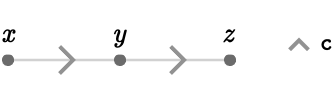#### Non-example

Imagine we try to define a path homomorphism $$\functionSignature{\function{ \gamma }}{\pathGroupoid{\quiver{Q}}}{\pathGroupoid{\quiver{R}}}$$, where we initially define its behavior on two particular paths $$\gbform{\path{P_1}},\rbform{\path{P_2}}$$ in $$\quiver{Q}$$: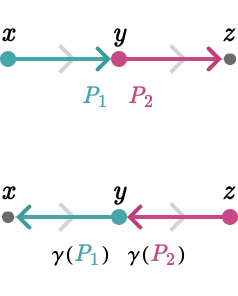There is no path homomorphism that behaves like this, because while we can form the composition $$\pathCompose{\gbform{\path{P_1}}}{\rbform{\path{P_2}}} = \rgform{\path{P_3}}$$, we cannot compose their images under $$\function{ \gamma }$$: $$\pathCompose{\function{ \gamma }(\gbform{\path{P_1}})}{\function{ \gamma }(\rbform{\path{P_2}})} = \nullElement$$, as the head of $$\function{ \gamma }(\gbform{\path{P_1}})$$ is not equal to the tail of $$\function{ \gamma }(\rbform{\path{P_2}})$$.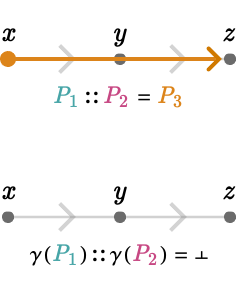#### Example

Now let us consider the map $$\functionSignature{\pathHomomorphism{ \rho }}{\pathGroupoid{\quiver{Q}}}{\pathGroupoid{\quiver{R}}}$$, illustrated below for paths $$\gbform{\path{P_1}},\rbform{\path{P_2}},\rgform{\path{P_3}}$$ as well as the empty paths $$\idElem{\vert{x}} = \paren{\pathWord{\vert{x}}{\emptyWord{}}{\vert{x}}},\idElem{\vert{y}} = \paren{\pathWord{\vert{y}}{\emptyWord{}}{\vert{y}}},\idElem{\vert{z}} = \paren{\pathWord{\vert{z}}{\emptyWord{}}{\vert{z}}}$$.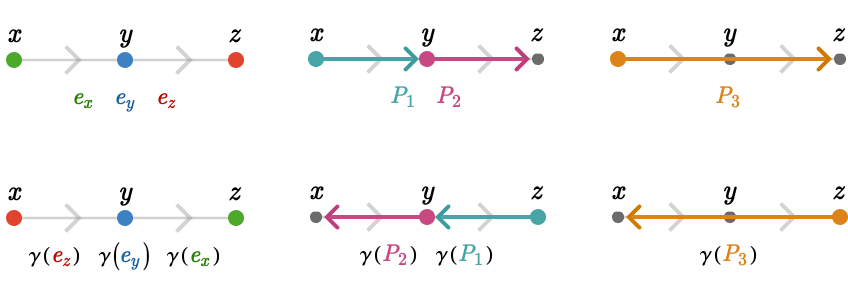This map does satisfy compatibility, making it a path homomorphism. Intuitively, $$\graphHomomorphism{ \gamma }$$ "reflects paths in the left-right axis" of the line quiver.

## Path tables

We can also visualize the behavior of above map as a path table showing the pairing of $$\path{P}$$ and $$\function{ \gamma }(\path{P})$$ for several paths $$\elemOf{\path{P}}{\pathList(\quiver{Q})}$$: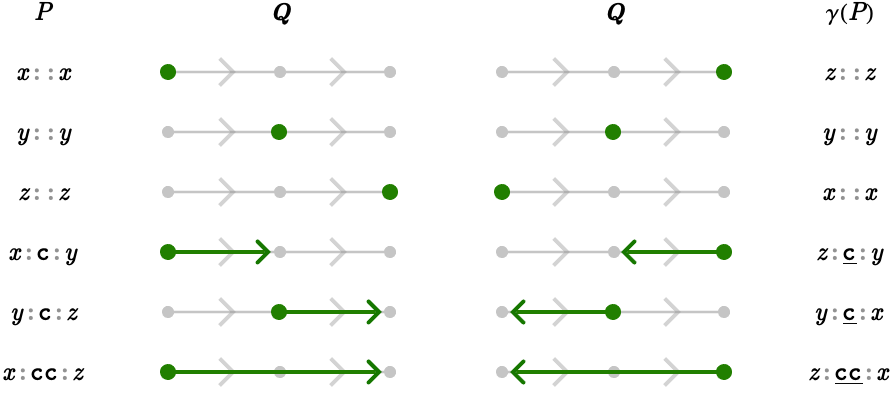To avoid having to illustrate $$\graphHomomorphism{ \gamma }$$ on every possible path, we can exploit the fact that $$\graphHomomorphism{ \gamma }$$ must preserve path reversal. The (undepicted) value of $$\function{ \gamma }(\pathWord{\vert{z}}{\word{\ncard{c}}{\ncard{c}}}{\vert{z}})$$ is implied by the (depicted) value $$\function{ \gamma }(\pathWord{\vert{x}}{\word{\card{c}}{\card{c}}}{\vert{z}})$$:

\begin{aligned} \function{ \gamma }(\pathReverse{\path{P}})&= \pathReverse{\function{ \gamma }(\path{P})}\\ \function{ \gamma }(\pathWord{\vert{z}}{\word{\ncard{c}}{\ncard{c}}}{\vert{x}})&= \pathReverse{\paren{\function{ \gamma }(\pathWord{\vert{x}}{\word{\card{c}}{\card{c}}}{\vert{z}})}}\\ &= \pathReverse{\paren{\pathWord{\vert{z}}{\word{\ncard{c}}{\ncard{c}}}{\vert{x}}}}\\ &= \pathWord{\vert{x}}{\word{\card{c}}{\card{c}}}{\vert{z}}\end{aligned}

This symmetry implies the remaining paths: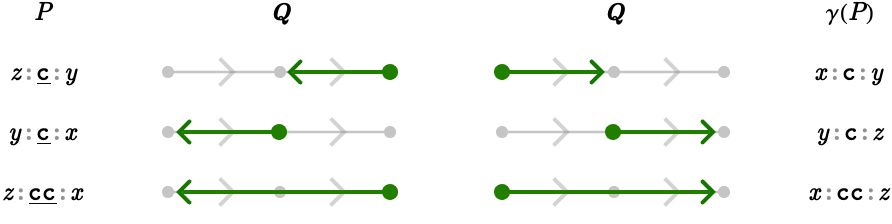Since these pairs are implied, we need not show these mappings in path tables.

#### Example: zero homomorphisms

Here we provide another example of a homomorphism, a zero homomorphism that sends every path to an empty path: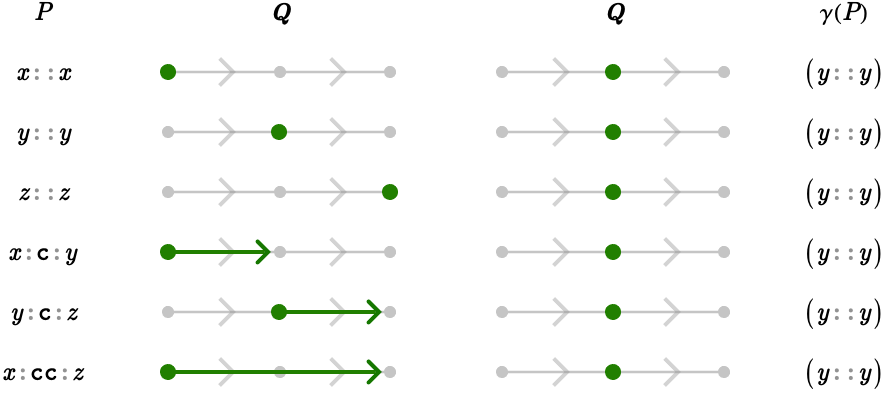There are three such possible zero homorphisms, because we can choose to send paths to the empty path on any of the three vertices in $$\quiver{Q}$$.

#### Example: partial zero homomorphisms

We can also construct path homomorphisms that "zero" some paths but not all paths. For example, we can zero the portion of any path that is "left" of the central vertex, $$\vert{y}$$: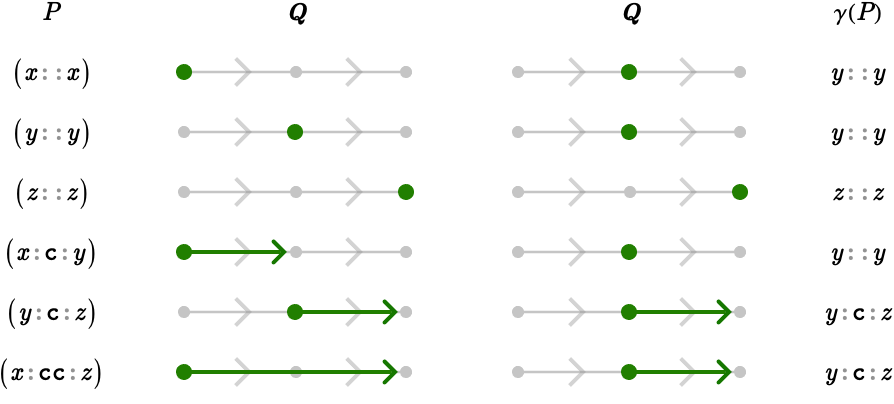#### Example: maps between two different quivers

So far, we've considered path represents from a single quiver to itself. Now let's consider a path homomorphism $$\functionSignature{\function{ \gamma }}{\pathGroupoid{\quiver{Q}}}{\pathGroupoid{\quiver{R}}}$$ between a line quiver $$\quiver{Q} = \bindCards{\subSize{\lineQuiver }{2}}{\rform{\card{r}}}$$, and a different quiver $$\quiver{R}$$ on cardinals $$\gform{\card{g}},\bform{\card{b}}$$: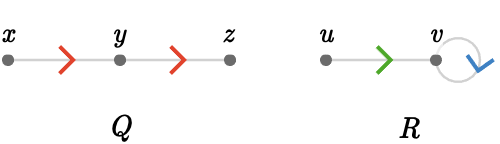Here we show the path table for $$\function{ \gamma }$$: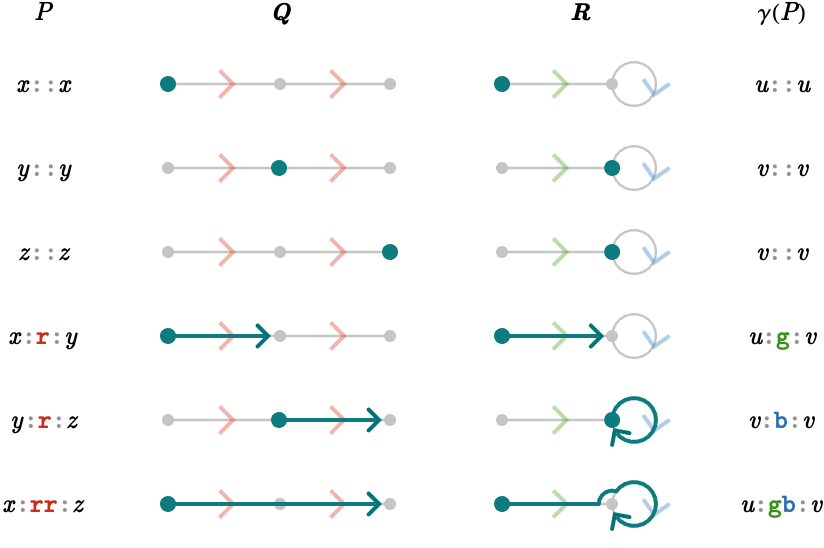## Images of edges

Let us consider the full path table for the homomorphism we just defined: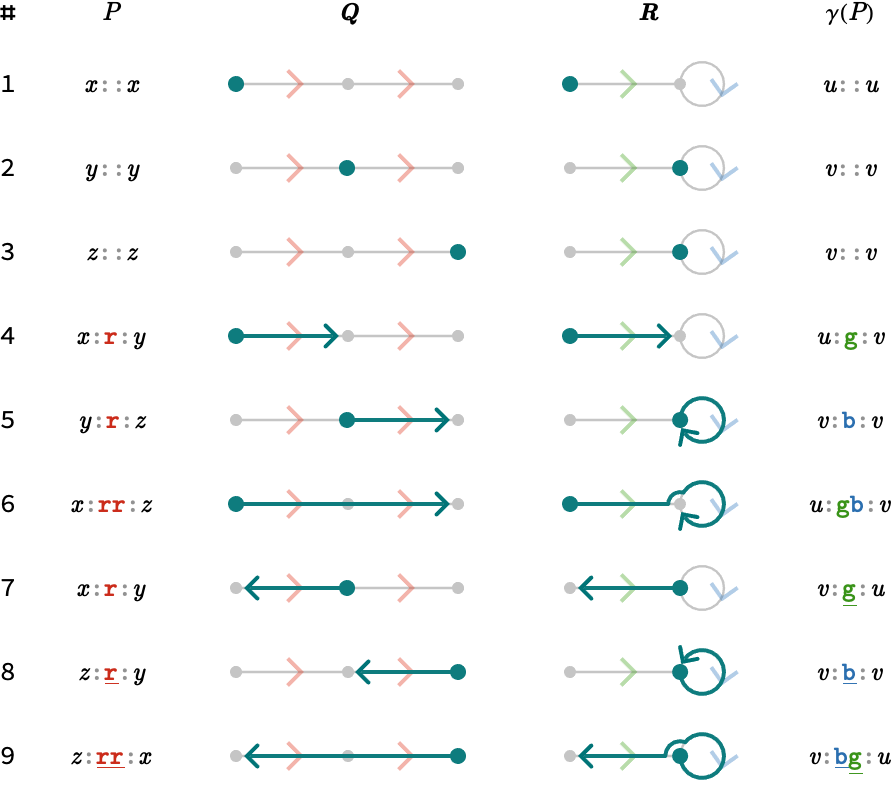This path table is obviously highly redundant, since many of the rows are implied by others:

• Rows 7, 8, and 9 are implied by 4, 5, and 6 via path reversal: $$\function{ \gamma }(\pathReverse{\path{P}}) = \pathReverse{\function{ \gamma }(\path{P})}$$.

• Rows 1, 2, and 3, which describe where to send empty paths on vertices $$\vert{x},\vert{y},\vert{z}$$, can be extracted from the heads (or tails) of the images of any paths that start (or end) at these vertices, e.g. rows 4 and 5.

• Row 6 depicts the image of path $$\paren{\pathWord{\vert{x}}{\word{\rform{\card{r}}}{\rform{\card{r}}}}{\vert{z}}}$$, but this path can be represented as the composition $$\pathCompose{\paren{\pathWord{\vert{x}}{\word{\rform{\card{r}}}}{\vert{y}}}}{\paren{\pathWord{\vert{y}}{\word{\rform{\card{r}}}}{\vert{z}}}}$$, and hence is determined by rows 4 and 5 (and compatibity).

All in all, only rows 4 and 5 are necessary to fully describe the behavior of $$\function{ \gamma }$$: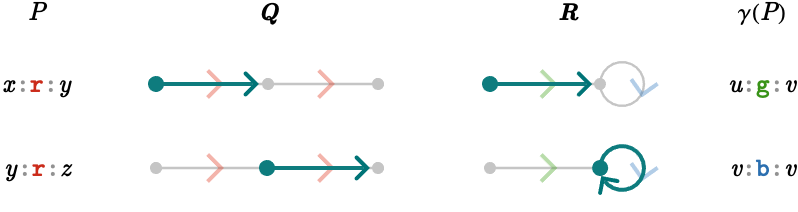But these are just the images of the edges of $$\quiver{Q}$$!

### Summary

We can see that the behavior of a path homomorphism $$\functionSignature{\function{ \gamma }}{\pathGroupoid{\quiver{Q}}}{\pathGroupoid{\quiver{R}}}$$ is uniquely determined by a choice of path $$\elemOf{\function{ \gamma }(\pathWord{\vert{x}}{\word{\card{c}}}{\vert{y}})}{\pathList(\quiver{Q})}$$ for every edge $$\elemOf{\tde{\vert{x}}{\vert{y}}{\card{c}}}{\edgeList(\quiver{Q})}$$. The behavior of $$\function{ \gamma }$$ on longer paths in $$\quiver{Q}$$ is then determined by composing the images of the sequence of edges in the path:

$\function{ \gamma }(\pathWord{\vert{x}}{\word{\card{\card{c}_1}}{\card{\card{c}_2}}{\card{\ellipsis }}{\card{\card{c}_{\sym{n}}}}}{\vert{z}}) = \pathCompose{\function{ \gamma }(\pathWord{\vert{x}}{\word{\card{\card{c}_1}}}{\blank})}{\function{ \gamma }(\pathWord{\blank}{\word{\card{\card{c}_2}}}{\blank})}{\ellipsis }{\function{ \gamma }(\pathWord{\blank}{\word{\card{\card{c}_{\sym{n}}}}}{\vert{z}})}$

The only requirement we must impose on these choices for individual edges is that the images of the edges can be composed in $$\quiver{R}$$ when two edges are head-to-tail in $$\quiver{Q}$$:

$\pathCompose{\function{ \gamma }(\pathWord{\vert{x}}{\word{\card{c}}}{\vert{y}})}{\function{ \gamma }(\pathWord{\vert{y}}{\word{\card{d}}}{\vert{z}})} \neq \nullElement$

## Lengthening and shortening

To get a further feeling for what kind of homomorphisms are possible, consider the following two graphs $$\quiver{Q} = \bindCards{\subSize{\lineQuiver }{3}}{\rform{\card{r}}}$$ and $$\path{R} = \bindCards{\subSize{\lineQuiver }{4}}{\rform{\card{r}}}$$: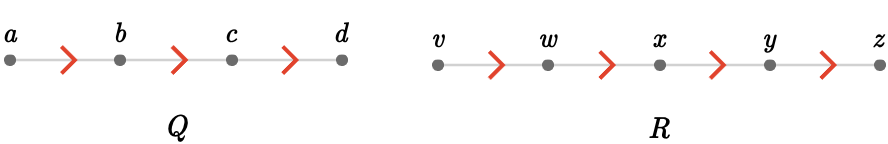#### Lengthening

Our path homomorphism $$\functionSignature{\function{ \gamma }}{\pathGroupoid{\quiver{Q}}}{\pathGroupoid{\quiver{R}}}$$ is defined by the following path table: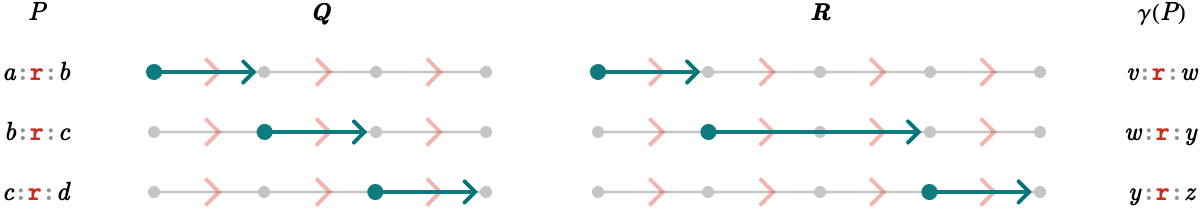Our homomorphism $$\function{ \gamma }$$ does a "jump" when traversing the middle edge in $$\quiver{Q}$$. We say it is a path lengthening, because there is at least one path whose image under $$\function{ \gamma }$$ is longer than the path:

$\existsForm{\path{P}}{\length(\graphHomomorphism{ \gamma }(\path{P}))>\length(\path{P})}$

This is equivalent to there being at least one edge that is sent to a path longer than 1:

$\existsForm{\elemOf{\edge{e}}{\edgeList(\quiver{Q})}}{\length(\graphHomomorphism{ \gamma }(\edge{e}))>1}$

We say a path homomorphism $$\function{ \gamma }$$ is $$\sym{k}$$-Lipschitz if the lengths of images of edges under $$\function{ \gamma }$$ are bounded above by $$\sym{k}$$:

$\forAllForm{\elemOf{\edge{e}}{\edgeList(\quiver{Q})}}{\length(\graphHomomorphism{ \gamma }(\edge{e})) \le \sym{k}}$

#### Shortening

Let us now consider a path homomorphism $$\functionSignature{\function{ \gamma }}{\pathGroupoid{\quiver{R}}}{\pathGroupoid{\quiver{Q}}}$$ with the opposite feature: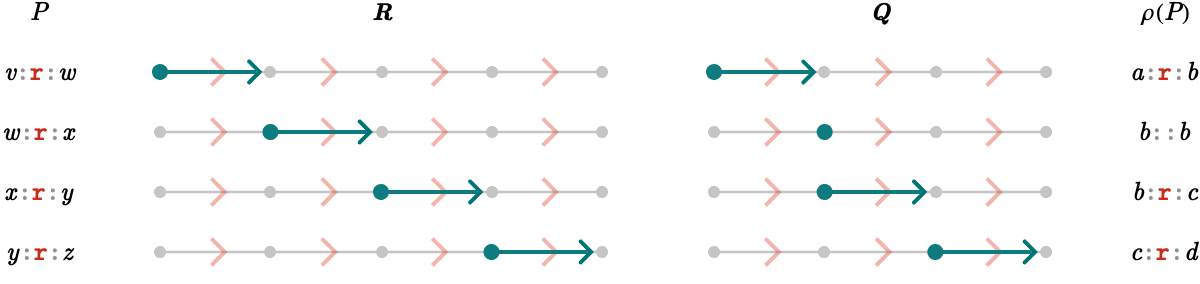Our homomorphism $$\pathHomomorphism{ \rho }$$ now "shortens" a particular edge, namely $$\paren{\pathWord{\vert{w}}{\word{\rform{\card{r}}}}{\vert{x}}}$$, sending it to $$\pathHomomorphism{ \rho }(\pathWord{\vert{w}}{\word{\rform{\card{r}}}}{\vert{x}}) = \paren{\pathWord{\vert{b}}{\emptyWord{}}{\vert{b}}}$$. Any longer paths that pass through this edge will similarly be shortened.

We say $$\pathHomomorphism{ \rho }$$ is a path shortening, because there is at least one path whose image under $$\function{ \gamma }$$ is shorter than the path:

$\existsForm{\path{P}}{\length(\graphHomomorphism{ \gamma }(\path{P}))<\length(\path{P})}$

This is equivalent to there being at least one edge that is sent to the empty path:

$\existsForm{\elemOf{\edge{e}}{\edgeList(\quiver{Q})}}{\length(\graphHomomorphism{ \gamma }(\edge{e})) = 0}$

### Composition

We can of course compose these path homomorphisms, in either order.

First, let's examine $$\functionSignature{\function{\paren{\functionComposition{\pathHomomorphism{ \rho }\functionCompositionSymbol \function{ \gamma }}}}}{\quiver{Q}}{\quiver{Q}}$$, which is $$\functionSignature{\pathHomomorphism{ \rho }}{\quiver{R}}{\quiver{Q}}$$ applied to the result of $$\functionSignature{\function{ \gamma }}{\quiver{Q}}{\quiver{R}}$$.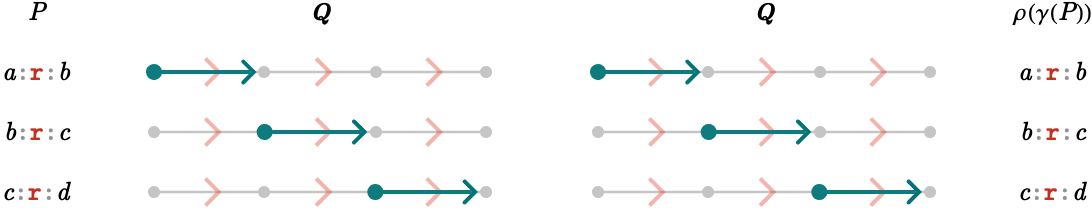This is the identity path homomorphism on $$\quiver{Q}$$.

Now let's examine $$\functionSignature{\function{\paren{\functionComposition{\function{ \gamma }\functionCompositionSymbol \pathHomomorphism{ \rho }}}}}{\quiver{R}}{\quiver{R}}$$, which is $$\functionSignature{\function{ \gamma }}{\quiver{Q}}{\quiver{R}}$$ applied to the result of $$\functionSignature{\pathHomomorphism{ \rho }}{\quiver{R}}{\quiver{Q}}$$: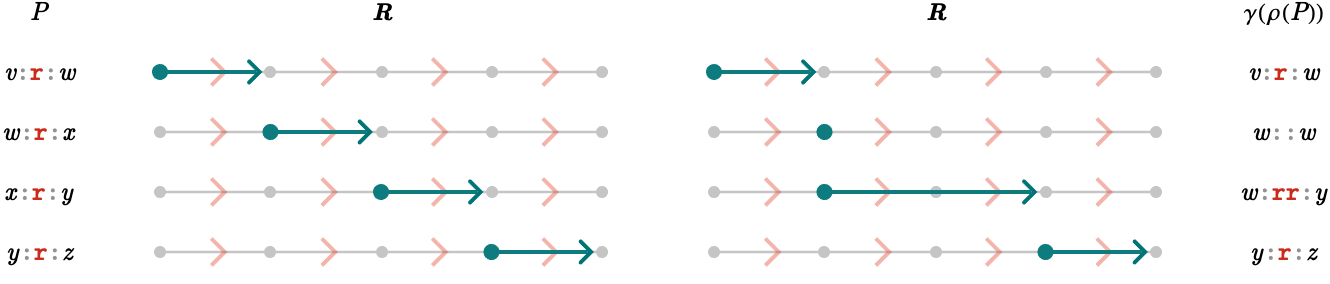This is certainly not the identity path homomorphism, and is both a shortening and a lengthing.

### Length preserving

We say a path homomorphism $$\function{ \gamma }$$ is length preserving if it is neither a shortening or a lengthening. This is equivalent to saying that the length of the image of every edge edge is exactly 1:

$\forAllForm{\elemOf{\edge{e}}{\edgeList(\quiver{Q})}}{\length(\graphHomomorphism{ \gamma }(\edge{e})) = 1}$

A length preserving homomorphism $$\functionSignature{\function{ \gamma }}{\pathGroupoid{\quiver{Q}}}{\pathGroupoid{\quiver{R}}}$$ map edges of $$\quiver{Q}$$ to oriented edges of $$\quiver{R}$$: a directed edge of $$\quiver{Q}$$ can be sent to a directed edge of $$\quiver{R}$$, but in a sense opposite to its ordinary orientation. The "horizontal reflection" homomorphism on $$\bindCards{\subSize{\lineQuiver }{2}}{\rform{\card{r}}}$$ we considered previously was of such a type.

## Path homomorphisms into the line quiver

For any quiver $$\quiver{Q}$$ there is a particular family of path homomorphisms that is particularly easy to characterize: the path homomorphisms onto the line quiver $$\subSize{\lineQuiver }{ \infty }$$.

To construct these, let us first define an integer vertex field to be a function $$\functionSignature{\function{f}}{\vertexList(\quiver{Q})}{\mathbb{Z}}$$ that assigns to each vertex of $$\quiver{Q}$$ an integer.

Below we show an example integer vertex field on the quiver $$\quiver{Q} = \subSize{\squareQuiver }{2}$$, where the values of $$\function{f}$$ are displayed beside each vertex: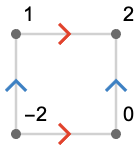We will now interpret the value $$\function{f}(\vert{v})$$ as determining a vertex of the line quiver $$\bindCards{\subSize{\lineQuiver }{ \infty }}{\gform{\card{g}}}$$:Therefore, $$\function{f}$$ induces part of the behavior of a path homomorphism $$\pathHomomorphism{ \rho }$$, specifically, the behavior of the empty paths of $$\quiver{Q}$$:

$\pathHomomorphism{ \rho }(\pathWord{\vert{v}}{\emptyWord{}}{\vert{v}})\defEqualSymbol \pathWord{\function{f}(\vert{v})}{\emptyWord{}}{\function{f}(\vert{v})}$

We now need only define $$\pathHomomorphism{ \rho }$$ on the edges of $$\quiver{Q}$$. To do this, we note that for any edge $$\elemOf{\tde{\vert{u}}{\vert{v}}{\card{c}}}{\edgeList(\quiver{Q})}$$, we are forced by compatibility to send the corresponding path $$\paren{\pathWord{\vert{u}}{\word{\card{c}}}{\vert{v}}}$$ to some path in $$\subSize{\lineQuiver }{ \infty }$$ with tail vertex $$\function{f}(\vert{u})$$ and head vertex $$\function{f}(\vert{v})$$. However, there is only one path in $$\subSize{\lineQuiver }{ \infty }$$between any two given vertices, and hence we have no choice in defining the remaining behavior of $$\pathHomomorphism{ \rho }$$.

The formal definition of $$\pathHomomorphism{ \rho }$$ then is as follows:

$\pathHomomorphism{ \rho }(\pathWord{\vert{u}}{\word{\card{c}}}{\vert{v}})\defEqualSymbol \paren{\pathWord{\function{f}(\vert{u})}{\repeatedPower{\word{\gform{\card{g}}}}{\function{f}(\vert{v}) - \function{f}(\vert{u})}}{\function{f}(\vert{v})}}$

We show part of the path table to make the idea clear: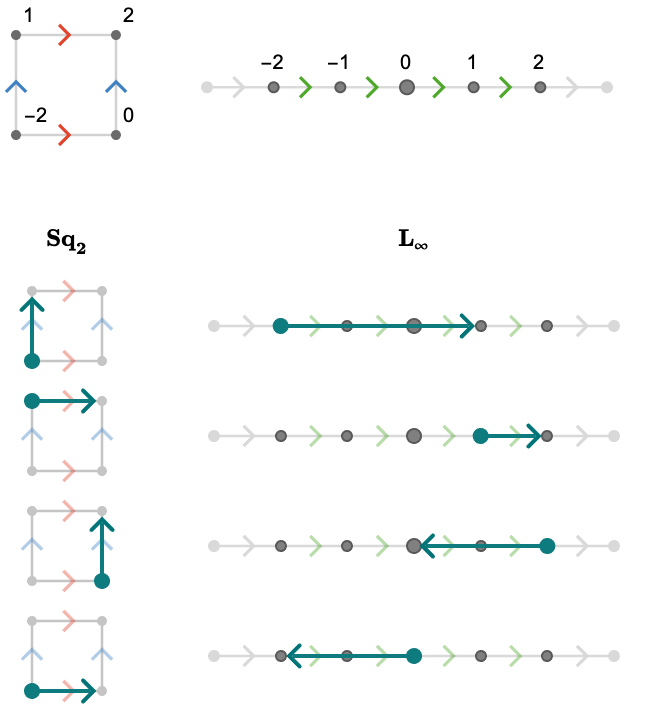Notice how the image of each edge under $$\pathHomomorphism{ \rho }$$ encodes the value of $$\function{f}$$ at the head and tail of the edge, and the cardinal content of the path encodes the difference between these values. In Path calculus we’ll develop a more formal approach to this kind of gradient-like operation.

The converse direction is trivally true: any path homomorphism into the line quiver gives us an integer vertex field, by simply numbering the vertices of the line quiver and taking pre-images of empty paths.

Therefore, we have that there is a bijection between integer vertex fields and path homomorphisms into the line quiver.

#### Connected quivers

We have just seen that a function $$\functionSignature{\function{f}}{\vertexList(\quiver{Q})}{\vertexList(\subSize{\lineQuiver }{ \infty })}$$ induces a unique path homomorphism $$\functionSignature{\pathHomomorphism{ \rho }}{\quiver{Q}}{\subSize{\lineQuiver }{ \infty }}$$, defining $$\pathHomomorphism{ \rho }(\de{\vert{u}}{\vert{v}})$$ to be the unique path in $$\subSize{\lineQuiver }{ \infty }$$ between vertices $$\function{f}(\vert{u})$$ and $$\function{f}(\vert{v})$$.

We can quite easily generalize this construction, replacing the $$\subSize{\lineQuiver }{ \infty }$$ with an arbitrary connected quiver $$\quiver{R}$$. However, for a given function $$\functionSignature{\function{f}}{\vertexList(\quiver{Q})}{\vertexList(\quiver{R})}$$ there may be more than one path between $$\function{f}(\vert{u})$$ and $$\function{f}(\vert{v})$$; we may in fact pick any path in $$\pathList(\path{R},\function{f}(\vert{u}),\function{f}(\vert{v}))$$ to define the behavior of the path homomorphism on an edge $$\pathHomomorphism{ \rho }(\de{\vert{u}}{\vert{v}})$$. Hence, we no longer have a bijection – typically there will be an infinite number of homomorphisms corresponding to a choice of function $$\function{f}$$.

## Endomorphisms and automorphisms

#### Endomorphisms

A special role is played by the set of path homomorphisms $$\functionSignature{\pathHomomorphism{ \alpha }}{\pathGroupoid{\quiver{Q}}}{\pathGroupoid{\quiver{Q}}}$$ from a quiver $$\quiver{Q}$$ to itself. We'll write this set as $$\endomorphisms(\quiver{Q})$$. We will now attach the structure of a semigroup to this set. That is to say, we will define an associative binary operation that corresponds to the composition of homomorphisms.

Like all function compositions, path homomorphism composition is associative. But is the composition of two homomorphisms $$\functionComposition{\pathHomomorphism{ \alpha }\functionCompositionSymbol \pathHomomorphism{ \beta }}$$ itself a homomorphism? This is easily seen to be true:

\begin{aligned} \function{\paren{\functionComposition{\pathHomomorphism{ \alpha }\functionCompositionSymbol \pathHomomorphism{ \beta }}}}(\pathCompose{\path{P_1}}{\path{P_2}})&= \pathHomomorphism{ \alpha }(\pathHomomorphism{ \beta }(\pathCompose{\path{P_1}}{\path{P_2}}))\\ &= \pathHomomorphism{ \alpha }(\pathCompose{\pathHomomorphism{ \beta }(\path{P_1})}{\pathHomomorphism{ \beta }(\path{P_2})})\\ &= \pathCompose{\pathHomomorphism{ \alpha }(\pathHomomorphism{ \beta }(\path{P_1}))}{\pathHomomorphism{ \alpha }(\pathHomomorphism{ \beta }(\path{P_2}))}\\ &= \pathCompose{\function{\paren{\functionComposition{\pathHomomorphism{ \alpha }\functionCompositionSymbol \pathHomomorphism{ \beta }}}}(\path{P_1})}{\function{\paren{\functionComposition{\pathHomomorphism{ \alpha }\functionCompositionSymbol \pathHomomorphism{ \beta }}}}(\path{P_2})}\end{aligned}

Therefore, the set $$\endomorphisms(\quiver{Q})$$ is a semigroup. It would further be a group if path homomorphisms were invertible, but we have seen examples of path homomorphisms that, for example, send all paths to the empty path on a particular vertex.

#### Automorphisms

So how can we constrain path homomorphisms to be invertible? It will turn out to be enough to ask that they are merely surjective, so that every possible path is the image of at least one other path:

$\forAllForm{\path{P}}{\existsForm{\path{R}}{\pathHomomorphism{ \alpha }(\path{R}) = \path{P}}}$

Why does surjectivity imply injectivity? We prove this by contradiction. Assume that a surjective path homomorphism $$\pathHomomorphism{ \alpha }$$ is not injective, so that:

$\existsForm{\path{P},\primed{\path{P}},\path{P} \neq \primed{\path{P}}}{\pathHomomorphism{ \alpha }(\path{P}) = \pathHomomorphism{ \alpha }(\primed{\path{P}})}$

If we decompose $$\path{P}$$ and $$\primed{\path{P}}$$ into sequences of individual 1-paths, we must obtain at least one pair $$\paren{\pathWord{\vert{u}}{\word{\card{c}}}{\vert{v}}},\paren{\pathWord{\vert{u}}{\word{\card{\primed{c}}}}{\primed{\vert{v}}}}$$ such that $$\pathHomomorphism{ \alpha }(\pathWord{\vert{u}}{\word{\card{c}}}{\vert{v}}) = \pathHomomorphism{ \alpha }(\pathWord{\vert{u}}{\word{\card{\primed{c}}}}{\primed{\vert{v}}})$$ but $$\vert{v} \neq \primed{\vert{v}}$$.## Affine path homomorphisms

You may have noticed that the discussion of path homomorphisms of quivers has not relied in any way on the cardinal structure of the quivers. In fact, everything we have said is applicable also to ordinary directed graphs and their path groupoids.

#### Word automorphisms

Recall that word group $$\wordGroup{\quiver{Q}}$$ is the free group on the cardinals of $$\quiver{Q}$$.

We now consider a group homomorphism $$\functionSignature{\groupHomomorphism{ \phi }}{\wordGroupSymbol }{\wordGroupSymbol }$$, or automorphism as such homomorphisms are called. We can think of such a homomorphism $$\groupHomomorphism{ \phi }$$ as a way of "rewriting" words as other words, letter-by-letter.

Let's consider an example homomorphism on a quiver with cardinals $$\cardinalList(\quiver{Q}) = \list{\rform{\card{r}},\gform{\card{g}},\bform{\card{b}}}$$. We define $$\groupHomomorphism{ \phi }$$ as:

$\groupHomomorphism{ \phi } = \groupWordRewriting{\rewritingRule{\word{\rform{\card{r}}}}{\word{\gform{\card{g}}}{\rform{\card{r}}}},\rewritingRule{\word{\gform{\card{g}}}}{\word{1}},\rewritingRule{\word{\bform{\card{b}}}}{\word{\rform{\ncard{r}}}}}$

The empty word has no letters to rewrite, so it remains the empty word:

$\groupHomomorphism{ \phi }(\card{1}) = \card{1}$

The words with one letter are rewritten as given in the definition of $$\groupHomomorphism{ \phi }$$. Because $$\groupHomomorphism{ \phi }$$ is a group homomorphism, it must replace the inverses of these cardinals with the inverses of the corresponding right-hand-sides:

$\begin{csarray}{rclrcl}{accicci} \groupHomomorphism{ \phi }(\rform{\card{r}}) & = & \word{\gform{\card{g}}}{\rform{\card{r}}} & \groupHomomorphism{ \phi }(\rform{\inverted{\card{r}}}) & = & \word{\rform{\ncard{r}}}{\gform{\ncard{g}}}\\ \groupHomomorphism{ \phi }(\gform{\card{g}}) & = & \card{1} & \groupHomomorphism{ \phi }(\gform{\inverted{\card{g}}}) & = & \card{1}\\ \groupHomomorphism{ \phi }(\bform{\card{b}}) & = & \rform{\inverted{\card{r}}} & \groupHomomorphism{ \phi }(\bform{\inverted{\card{b}}}) & = & \rform{\card{r}} \end{csarray}$

Longer words are rewritten left-to-right, since this is how a group homomorphism to $$\wordGroupSymbol$$ must work. Here we show a few examples of rewriting of longer words:

$\begin{array}{rclcl} \groupHomomorphism{ \phi }(\word{\rform{\card{r}}}{\rform{\card{r}}}) & = & \groupHomomorphism{ \phi }(\rform{\card{r}})\iGmult \groupHomomorphism{ \phi }(\rform{\card{r}}) & = & \word{\gform{\card{g}}}{\rform{\card{r}}}{\gform{\card{g}}}{\rform{\card{r}}}\\ \groupHomomorphism{ \phi }(\word{\rform{\ncard{r}}}{\rform{\ncard{r}}}) & = & \groupHomomorphism{ \phi }(\rform{\inverted{\card{r}}})\iGmult \groupHomomorphism{ \phi }(\rform{\inverted{\card{r}}}) & = & \word{\rform{\ncard{r}}}{\gform{\ncard{g}}}{\rform{\ncard{r}}}{\gform{\ncard{g}}}\\ \groupHomomorphism{ \phi }(\word{\gform{\card{g}}}{\gform{\card{g}}}) & = & \groupHomomorphism{ \phi }(\gform{\card{g}})\iGmult \groupHomomorphism{ \phi }(\gform{\card{g}}) = \concat{\card{1}\,\card{1}} & = & \card{1}\\ \groupHomomorphism{ \phi }(\word{\bform{\card{b}}}{\bform{\card{b}}}) & = & \groupHomomorphism{ \phi }(\bform{\card{b}})\iGmult \groupHomomorphism{ \phi }(\bform{\card{b}}) & = & \word{\rform{\ncard{r}}}{\rform{\ncard{r}}}\\ \groupHomomorphism{ \phi }(\word{\rform{\card{r}}}{\bform{\card{b}}}) & = & \groupHomomorphism{ \phi }(\rform{\card{r}})\iGmult \groupHomomorphism{ \phi }(\bform{\card{b}}) = \concat{\word{\gform{\card{g}}}{\rform{\card{r}}}\,\rform{\inverted{\card{r}}}} & = & \gform{\card{g}}\\ \groupHomomorphism{ \phi }(\word{\rform{\card{r}}}{\gform{\card{g}}}{\bform{\card{b}}}) & = & \groupHomomorphism{ \phi }(\rform{\card{r}})\iGmult \groupHomomorphism{ \phi }(\gform{\card{g}})\iGmult \groupHomomorphism{ \phi }(\bform{\card{b}}) = \concat{\word{\gform{\card{g}}}{\rform{\card{r}}}\,\card{1}\,\rform{\inverted{\card{r}}}} & = & \gform{\card{g}}\\ \groupHomomorphism{ \phi }(\word{\rform{\card{r}}}{\gform{\card{g}}}{\bform{\ncard{b}}}) & = & \groupHomomorphism{ \phi }(\rform{\card{r}})\iGmult \groupHomomorphism{ \phi }(\gform{\card{g}})\iGmult \groupHomomorphism{ \phi }(\bform{\inverted{\card{b}}}) = \concat{\word{\gform{\card{g}}}{\rform{\card{r}}}\,\card{1}\,\rform{\card{r}}} & = & \word{\gform{\card{g}}}{\rform{\card{r}}}{\rform{\card{r}}} \end{array}$

Repeating the story of the composition of path homomorphisms, we can now form the endomorphism semigroup $$\endomorphisms(\wordGroupSymbol )$$ of word homomorphisms.

### Automorphism group

If we want the group $$\automorphisms(\wordGroupSymbol )$$ rather than the semigroup $$\endomorphisms(\wordGroupSymbol )$$, we need to limit ourselves to invertible word homomoprhisms.

As a first step to characterizing these, observe that any signed permutation of the set of cardinals gives an invertible homomorphism. Signed permutations are permutations that can additionally invert each element. Since the number of permutations of $$\sym{n}$$ elements is $$\factorial{\sym{n}}$$, and the number of inverse patterns is $$\power{2}{\sym{n}}$$, there are $$\factorial{\sym{n}} \, \power{2}{\sym{n}}$$ signed permutations.

For example, the 8 possible signed permutations on $$\list{\rform{\card{r}},\bform{\card{b}}}$$ are $$\list{\tuple{\rform{\card{r}},\bform{\card{b}}},\tuple{\inverted{\rform{\card{r}}},\bform{\card{b}}},\tuple{\rform{\card{r}},\inverted{\bform{\card{b}}}},\tuple{\inverted{\rform{\card{r}}},\inverted{\bform{\card{b}}}},\tuple{\bform{\card{b}},\rform{\card{r}}},\tuple{\inverted{\bform{\card{b}}},\rform{\card{r}}},\tuple{\bform{\card{b}},\inverted{\rform{\card{r}}}},\tuple{\inverted{\bform{\card{b}}},\inverted{\rform{\card{r}}}}}$$, yielding the following word automorphisms:

$\begin{array}{c} \groupWordRewriting{\rewritingRule{\rform{\card{r}}}{\rform{\card{r}}},\rewritingRule{\bform{\card{b}}}{\bform{\card{b}}}}\\ \groupWordRewriting{\rewritingRule{\rform{\card{r}}}{\rform{\inverted{\card{r}}}},\rewritingRule{\bform{\card{b}}}{\bform{\card{b}}}}\\ \groupWordRewriting{\rewritingRule{\rform{\card{r}}}{\rform{\card{r}}},\rewritingRule{\bform{\card{b}}}{\bform{\inverted{\card{b}}}}}\\ \groupWordRewriting{\rewritingRule{\rform{\card{r}}}{\rform{\inverted{\card{r}}}},\rewritingRule{\bform{\card{b}}}{\bform{\inverted{\card{b}}}}}\\ \groupWordRewriting{\rewritingRule{\rform{\card{r}}}{\bform{\card{b}}},\rewritingRule{\bform{\card{b}}}{\rform{\card{r}}}}\\ \groupWordRewriting{\rewritingRule{\rform{\card{r}}}{\bform{\inverted{\card{b}}}},\rewritingRule{\bform{\card{b}}}{\rform{\card{r}}}}\\ \groupWordRewriting{\rewritingRule{\rform{\card{r}}}{\bform{\card{b}}},\rewritingRule{\bform{\card{b}}}{\rform{\inverted{\card{r}}}}}\\ \groupWordRewriting{\rewritingRule{\rform{\card{r}}}{\bform{\inverted{\card{b}}}},\rewritingRule{\bform{\card{b}}}{\rform{\inverted{\card{r}}}}} \end{array}$

Moving to the set $$\list{\rform{\card{r}},\gform{\card{g}},\bform{\card{b}}}$$ yields 48 signed permutations: $$\tuple{\rform{\card{r}},\gform{\card{g}},\bform{\card{b}}},\tuple{\inverted{\rform{\card{r}}},\gform{\card{g}},\bform{\card{b}}},\tuple{\rform{\card{r}},\inverted{\gform{\card{g}}},\bform{\card{b}}},\tuple{\rform{\card{r}},\gform{\card{g}},\inverted{\bform{\card{b}}}}$$, $$\ellipsis$$, $$\tuple{\inverted{\bform{\card{b}}},\inverted{\gform{\card{g}}},\rform{\card{r}}},\tuple{\inverted{\bform{\card{b}}},\gform{\card{g}},\inverted{\rform{\card{r}}}},\tuple{\bform{\card{b}},\inverted{\gform{\card{g}}},\inverted{\rform{\card{r}}}},\tuple{\inverted{\bform{\card{b}}},\inverted{\gform{\card{g}}},\inverted{\rform{\card{r}}}}$$, yielding 48 word automorphisms:

$\begin{array}{c} \groupWordRewriting{\rewritingRule{\rform{\card{r}}}{\rform{\card{r}}},\rewritingRule{\gform{\card{g}}}{\gform{\card{g}}},\rewritingRule{\bform{\card{b}}}{\bform{\card{b}}}}\\ \groupWordRewriting{\rewritingRule{\rform{\card{r}}}{\rform{\inverted{\card{r}}}},\rewritingRule{\gform{\card{g}}}{\gform{\card{g}}},\rewritingRule{\bform{\card{b}}}{\bform{\card{b}}}}\\ \groupWordRewriting{\rewritingRule{\rform{\card{r}}}{\rform{\card{r}}},\rewritingRule{\gform{\card{g}}}{\gform{\inverted{\card{g}}}},\rewritingRule{\bform{\card{b}}}{\bform{\card{b}}}}\\ \groupWordRewriting{\rewritingRule{\rform{\card{r}}}{\rform{\card{r}}},\rewritingRule{\gform{\card{g}}}{\gform{\card{g}}},\rewritingRule{\bform{\card{b}}}{\bform{\inverted{\card{b}}}}}\\ \verticalEllipsis \\ \groupWordRewriting{\rewritingRule{\rform{\card{r}}}{\bform{\inverted{\card{b}}}},\rewritingRule{\gform{\card{g}}}{\gform{\inverted{\card{g}}}},\rewritingRule{\bform{\card{b}}}{\rform{\card{r}}}}\\ \groupWordRewriting{\rewritingRule{\rform{\card{r}}}{\bform{\inverted{\card{b}}}},\rewritingRule{\gform{\card{g}}}{\gform{\card{g}}},\rewritingRule{\bform{\card{b}}}{\rform{\inverted{\card{r}}}}}\\ \groupWordRewriting{\rewritingRule{\rform{\card{r}}}{\bform{\card{b}}},\rewritingRule{\gform{\card{g}}}{\gform{\inverted{\card{g}}}},\rewritingRule{\bform{\card{b}}}{\rform{\inverted{\card{r}}}}}\\ \groupWordRewriting{\rewritingRule{\rform{\card{r}}}{\bform{\inverted{\card{b}}}},\rewritingRule{\gform{\card{g}}}{\gform{\inverted{\card{g}}}},\rewritingRule{\bform{\card{b}}}{\rform{\inverted{\card{r}}}}} \end{array}$

Being obviously invertible, the signed permutations on $$\sym{n}$$ cardinals form a group. This group is known as the Coxeter group $$\group{B_{\sym{n}}}$$.

#### Lengthening automorphisms

This exhausts the word automorphisms that do not lengthen words. But it is not immediately obvious that they can lengthen word while still remaining invertible. Here we demonstrate one particular example and explain why it is invertible:

$\groupHomomorphism{ \phi } = \groupWordRewriting{\rewritingRule{\word{\rform{\card{r}}}}{\word{\rform{\card{r}}}{\gform{\ncard{g}}}},\rewritingRule{\word{\gform{\card{g}}}}{\word{\gform{\card{g}}}{\bform{\ncard{b}}}},\rewritingRule{\word{\bform{\card{b}}}}{\word{\bform{\card{b}}}}}$

To show invertibility, we need to explicitly construct words (of any length) that map to the single-cardinal words $$\list{\rform{\card{r}},\gform{\card{g}},\bform{\card{b}}}$$. Inverses of longer words can then be formed by composing these primitive inverses. To find these primitive inverses, we observe that:

$\begin{array}{rcccccl} \rform{\card{r}} & = & \concat{\word{\rform{\card{r}}}{\gform{\ncard{g}}}\,\word{\gform{\card{g}}}{\bform{\ncard{b}}}\,\bform{\card{b}}} & = & \concat{\groupHomomorphism{ \phi }(\rform{\card{r}})\,\groupHomomorphism{ \phi }(\gform{\card{g}})\,\groupHomomorphism{ \phi }(\bform{\card{b}})} & = & \groupHomomorphism{ \phi }(\word{\rform{\card{r}}}{\gform{\card{g}}}{\bform{\card{b}}})\\ \gform{\card{g}} & = & \concat{\word{\gform{\card{g}}}{\bform{\ncard{b}}}\,\bform{\card{b}}} & = & \concat{\groupHomomorphism{ \phi }(\gform{\card{g}})\,\groupHomomorphism{ \phi }(\bform{\card{b}})} & = & \groupHomomorphism{ \phi }(\word{\gform{\card{g}}}{\bform{\card{b}}})\\ \bform{\card{b}} & = & & & & = & \groupHomomorphism{ \phi }(\bform{\card{b}}) \end{array}$

Hence we have that $$\inverse{\groupHomomorphism{ \phi }} = \groupWordRewriting{\rewritingRule{\word{\rform{\card{r}}}}{\word{\rform{\card{r}}}{\gform{\card{g}}}{\bform{\card{b}}}},\rewritingRule{\word{\gform{\card{g}}}}{\word{\gform{\card{g}}}{\bform{\card{b}}}},\rewritingRule{\word{\bform{\card{b}}}}{\word{\bform{\card{b}}}}}$$.

More generally, characterizing the group structure of the automorphism group of the free group is tricky, with the most famous result being a presentation in terms of so-called Nielsen transformations, which we will not go into here.

#### Abelian word group

If we temporarily imagine the word group to be Abelian, in other words, if $$\word{\rform{\card{r}}}{\gform{\card{g}}} = \word{\gform{\card{g}}}{\rform{\card{r}}},\word{\gform{\card{g}}}{\bform{\card{b}}} = \word{\bform{\card{b}}}{\gform{\card{g}}}$$, etc., things become much easier. In this setting, we can represent a word homomorphism $$\groupHomomorphism{ \phi }$$ on $$\sym{n}$$ cardinals as an $$\sym{n}\times \sym{n}$$ integral matrix $$\matrix{M_{\groupHomomorphism{ \phi }}}$$ by choosing an ordering of the cardinals to serve as the basis . Here we depict four examples:

$\begin{csarray}{cccc}{aamam} \groupHomomorphism{ \phi } & \matrix{M_{\groupHomomorphism{ \phi }}} & \groupHomomorphism{ \phi } & \matrix{M_{\groupHomomorphism{ \phi }}}\\ & & & \\ \begin{array}{l} \langle \kern{2pt}\rewritingRule{\word{\rform{\card{r}}}}{\word{\rform{\card{r}}}},\\ \phantom{ \langle \kern{2pt}}\rewritingRule{\word{\gform{\card{g}}}}{\word{\gform{\card{g}}}},\\ \phantom{ \langle \kern{2pt}}\rewritingRule{\word{\bform{\card{b}}}}{\word{\bform{\card{b}}}}\kern{2pt} \rangle \end{array} & {\begin{pmatrix}1&0&0\\0&1&0\\0&0&1\end{pmatrix}} & \begin{array}{l} \langle \kern{2pt}\rewritingRule{\word{\rform{\card{r}}}}{\word{\rform{\card{r}}}{\gform{\card{g}}}{\bform{\card{b}}}},\\ \phantom{ \langle \kern{2pt}}\rewritingRule{\word{\gform{\card{g}}}}{\word{\gform{\card{g}}}{\bform{\card{b}}}},\\ \phantom{ \langle \kern{2pt}}\rewritingRule{\word{\bform{\card{b}}}}{\word{\bform{\card{b}}}}\kern{2pt} \rangle \end{array} & {\begin{pmatrix}1&1&1\\0&1&1\\0&0&1\end{pmatrix}}\\ & & & \\ \begin{array}{l} \langle \kern{2pt}\rewritingRule{\word{\rform{\card{r}}}}{\word{\card{1}}},\\ \phantom{ \langle \kern{2pt}}\rewritingRule{\word{\gform{\card{g}}}}{\word{\gform{\card{g}}}},\\ \phantom{ \langle \kern{2pt}}\rewritingRule{\word{\bform{\card{b}}}}{\word{\bform{\card{b}}}}\kern{2pt} \rangle \end{array} & {\begin{pmatrix}0&0&0\\0&1&0\\0&0&1\end{pmatrix}} & \begin{array}{l} \langle \kern{2pt}\rewritingRule{\word{\rform{\card{r}}}}{\word{\rform{\ncard{r}}}},\\ \phantom{ \langle \kern{2pt}}\rewritingRule{\word{\gform{\card{g}}}}{\word{\bform{\card{b}}}},\\ \phantom{ \langle \kern{2pt}}\rewritingRule{\word{\bform{\card{b}}}}{\word{\gform{\card{g}}}}\kern{2pt} \rangle \end{array} & {\begin{pmatrix}-1&0&0\\0&0&1\\0&1&0\end{pmatrix}} \end{csarray}$

Finding the inverse homomorphism is equivalent to inverting its integral matrix:

$\matrix{M_{\inverse{\groupHomomorphism{ \phi }}}} = \inverse{\matrix{M_{\groupHomomorphism{ \phi }}}}$

Even better, it is easily checked that an integral matrix is only invertible if its determinant is $$\pm 1$$, allowing us to characterize the automorphism group of Abelian word homomorphisms as the integral orthogonal group $$\orthogonalGroup{\sym{n}}{\ring{\mathbb{Z}}}$$.

## Visualization

We can visualize the action of various word homomorphisms on some familiar transitive quivers to gain intuition for their behavior.